# Interpolation splinethat coincides with a given function at given distinct points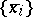. For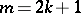one usually takes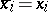,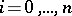, and since forthere still are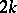free parameters, one prescribesadditional conditions atand, e.g.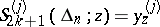,,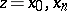, where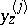are given numbers. If thelinearly depend on the given function, then the corresponding spline linearly depends on this function. Forone usually takes,and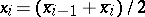,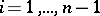, andadditional conditions are prescribed atand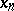. If the spline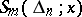has an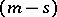-th continuous and an-st discontinuous derivative at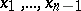, then for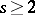the first-st derivatives of the spline are prescribed at these points, requiring them to coincide with the corresponding derivatives of the function to be interpolated. Interpolation- and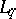-splines, as well as interpolation splines in several variables, have also been considered. Interpolation splines are used to approximate a function using its values on a grid. In contrast to interpolation polynomials, there exist matrices of nodes such that the interpolation splines converge to an arbitrary given continuous integrable function.